Travis-CI:# Usage Guide¶

This tutorial covers some basic usage patterns and best-practices to help you get started with Matplotlib.

## General Concepts¶

`matplotlib` has an extensive codebase that can be daunting to many new users. However, most of matplotlib can be understood with a fairly simple conceptual framework and knowledge of a few important points.

Plotting requires action on a range of levels, from the most general (e.g., ‘contour this 2-D array’) to the most specific (e.g., ‘color this screen pixel red’). The purpose of a plotting package is to assist you in visualizing your data as easily as possible, with all the necessary control – that is, by using relatively high-level commands most of the time, and still have the ability to use the low-level commands when needed.

Therefore, everything in matplotlib is organized in a hierarchy. At the top of the hierarchy is the matplotlib “state-machine environment” which is provided by the `matplotlib.pyplot` module. At this level, simple functions are used to add plot elements (lines, images, text, etc.) to the current axes in the current figure.

Note

Pyplot’s state-machine environment behaves similarly to MATLAB and should be most familiar to users with MATLAB experience.

The next level down in the hierarchy is the first level of the object-oriented interface, in which pyplot is used only for a few functions such as figure creation, and the user explicitly creates and keeps track of the figure and axes objects. At this level, the user uses pyplot to create figures, and through those figures, one or more axes objects can be created. These axes objects are then used for most plotting actions.

For even more control – which is essential for things like embedding matplotlib plots in GUI applications – the pyplot level may be dropped completely, leaving a purely object-oriented approach.

```# sphinx_gallery_thumbnail_number = 3
import matplotlib.pyplot as plt
import numpy as np
```

## Parts of a Figure¶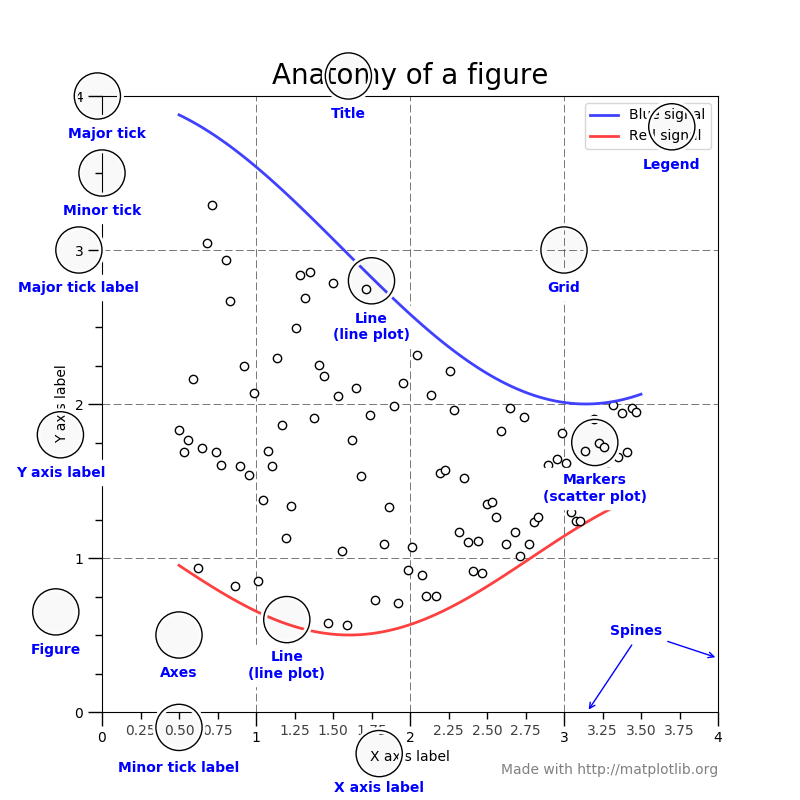### `Figure`¶

The whole figure. The figure keeps track of all the child `Axes`, a smattering of ‘special’ artists (titles, figure legends, etc), and the canvas. (Don’t worry too much about the canvas, it is crucial as it is the object that actually does the drawing to get you your plot, but as the user it is more-or-less invisible to you). A figure can have any number of `Axes`, but to be useful should have at least one.

The easiest way to create a new figure is with pyplot:

```fig = plt.figure()  # an empty figure with no axes
fig.suptitle('No axes on this figure')  # Add a title so we know which it is

fig, ax_lst = plt.subplots(2, 2)  # a figure with a 2x2 grid of Axes
```

### `Axes`¶

This is what you think of as ‘a plot’, it is the region of the image with the data space. A given figure can contain many Axes, but a given `Axes` object can only be in one `Figure`. The Axes contains two (or three in the case of 3D) `Axis` objects (be aware of the difference between Axes and Axis) which take care of the data limits (the data limits can also be controlled via set via the `set_xlim()` and `set_ylim()` `Axes` methods). Each `Axes` has a title (set via `set_title()`), an x-label (set via `set_xlabel()`), and a y-label set via `set_ylabel()`).

The `Axes` class and it’s member functions are the primary entry point to working with the OO interface.

### `Axis`¶

These are the number-line-like objects. They take care of setting the graph limits and generating the ticks (the marks on the axis) and ticklabels (strings labeling the ticks). The location of the ticks is determined by a `Locator` object and the ticklabel strings are formatted by a `Formatter`. The combination of the correct `Locator` and `Formatter` gives very fine control over the tick locations and labels.

### `Artist`¶

Basically everything you can see on the figure is an artist (even the `Figure`, `Axes`, and `Axis` objects). This includes `Text` objects, `Line2D` objects, `collection` objects, `Patch` objects … (you get the idea). When the figure is rendered, all of the artists are drawn to the canvas. Most Artists are tied to an Axes; such an Artist cannot be shared by multiple Axes, or moved from one to another.

## Types of inputs to plotting functions¶

All of plotting functions expect `np.array` or `np.ma.masked_array` as input. Classes that are ‘array-like’ such as `pandas` data objects and `np.matrix` may or may not work as intended. It is best to convert these to `np.array` objects prior to plotting.

For example, to convert a `pandas.DataFrame`

```a = pandas.DataFrame(np.random.rand(4,5), columns = list('abcde'))
a_asndarray = a.values
```

and to covert a `np.matrix`

```b = np.matrix([[1,2],[3,4]])
b_asarray = np.asarray(b)
```

## Coding Styles¶

When viewing this documentation and examples, you will find different coding styles and usage patterns. These styles are perfectly valid and have their pros and cons. Just about all of the examples can be converted into another style and achieve the same results. The only caveat is to avoid mixing the coding styles for your own code.

Note

Developers for matplotlib have to follow a specific style and guidelines. See The Matplotlib Developers’ Guide.

Of the different styles, there are two that are officially supported. Therefore, these are the preferred ways to use matplotlib.

For the pyplot style, the imports at the top of your scripts will typically be:

```import matplotlib.pyplot as plt
import numpy as np
```

Then one calls, for example, np.arange, np.zeros, np.pi, plt.figure, plt.plot, plt.show, etc. Use the pyplot interface for creating figures, and then use the object methods for the rest:

```x = np.arange(0, 10, 0.2)
y = np.sin(x)
fig = plt.figure()
ax.plot(x, y)
plt.show()
```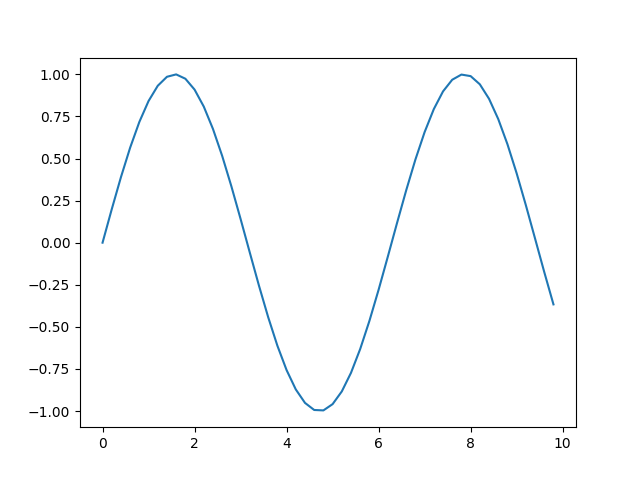So, why all the extra typing instead of the MATLAB-style (which relies on global state and a flat namespace)? For very simple things like this example, the only advantage is academic: the wordier styles are more explicit, more clear as to where things come from and what is going on. For more complicated applications, this explicitness and clarity becomes increasingly valuable, and the richer and more complete object-oriented interface will likely make the program easier to write and maintain.

Typically one finds oneself making the same plots over and over again, but with different data sets, which leads to needing to write specialized functions to do the plotting. The recommended function signature is something like:

```def my_plotter(ax, data1, data2, param_dict):
"""
A helper function to make a graph

Parameters
----------
ax : Axes
The axes to draw to

data1 : array
The x data

data2 : array
The y data

param_dict : dict
Dictionary of kwargs to pass to ax.plot

Returns
-------
out : list
"""
out = ax.plot(data1, data2, **param_dict)
return out

# which you would then use as:

data1, data2, data3, data4 = np.random.randn(4, 100)
fig, ax = plt.subplots(1, 1)
my_plotter(ax, data1, data2, {'marker': 'x'})
```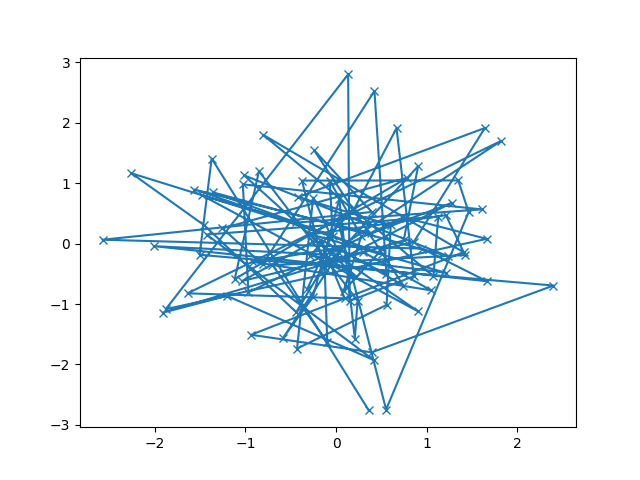or if you wanted to have 2 sub-plots:

```fig, (ax1, ax2) = plt.subplots(1, 2)
my_plotter(ax1, data1, data2, {'marker': 'x'})
my_plotter(ax2, data3, data4, {'marker': 'o'})
```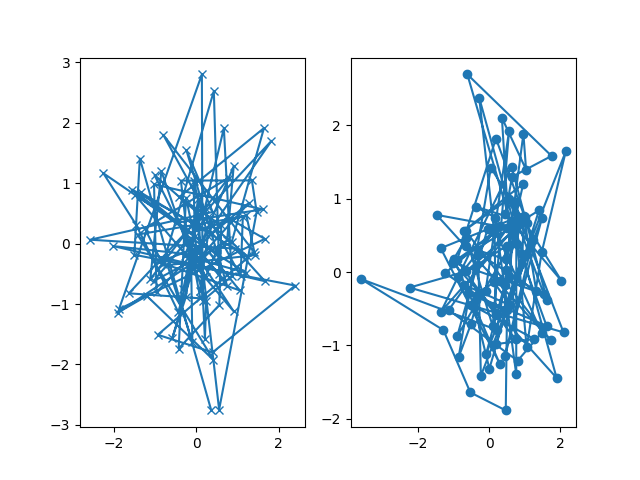Again, for these simple examples this style seems like overkill, however once the graphs get slightly more complex it pays off.

## Backends¶

### What is a backend?¶

A lot of documentation on the website and in the mailing lists refers to the “backend” and many new users are confused by this term. matplotlib targets many different use cases and output formats. Some people use matplotlib interactively from the python shell and have plotting windows pop up when they type commands. Some people embed matplotlib into graphical user interfaces like wxpython or pygtk to build rich applications. Others use matplotlib in batch scripts to generate postscript images from some numerical simulations, and still others in web application servers to dynamically serve up graphs.

To support all of these use cases, matplotlib can target different outputs, and each of these capabilities is called a backend; the “frontend” is the user facing code, i.e., the plotting code, whereas the “backend” does all the hard work behind-the-scenes to make the figure. There are two types of backends: user interface backends (for use in pygtk, wxpython, tkinter, qt4, or macosx; also referred to as “interactive backends”) and hardcopy backends to make image files (PNG, SVG, PDF, PS; also referred to as “non-interactive backends”).

There are four ways to configure your backend. If they conflict each other, the method mentioned last in the following list will be used, e.g. calling `use()` will override the setting in your `matplotlibrc`.

1. The `backend` parameter in your `matplotlibrc` file (see Customizing matplotlib):

```backend : WXAgg   # use wxpython with antigrain (agg) rendering
```
2. Setting the `MPLBACKEND` environment variable, either for your current shell or for a single script:

```> export MPLBACKEND="module://my_backend"
> python simple_plot.py

> MPLBACKEND="module://my_backend" python simple_plot.py
```

Setting this environment variable will override the `backend` parameter in any `matplotlibrc`, even if there is a `matplotlibrc` in your current working directory. Therefore setting `MPLBACKEND` globally, e.g. in your `.bashrc` or `.profile`, is discouraged as it might lead to counter-intuitive behavior.

3. If your script depends on a specific backend you can use the `use()` function:

```import matplotlib
matplotlib.use('PS')   # generate postscript output by default
```

If you use the `use()` function, this must be done before importing `matplotlib.pyplot`. Calling `use()` after pyplot has been imported will have no effect. Using `use()` will require changes in your code if users want to use a different backend. Therefore, you should avoid explicitly calling `use()` unless absolutely necessary.

Note

Backend name specifications are not case-sensitive; e.g., ‘GTKAgg’ and ‘gtkagg’ are equivalent.

With a typical installation of matplotlib, such as from a binary installer or a linux distribution package, a good default backend will already be set, allowing both interactive work and plotting from scripts, with output to the screen and/or to a file, so at least initially you will not need to use any of the methods given above.

If, however, you want to write graphical user interfaces, or a web application server (Matplotlib in a web application server), or need a better understanding of what is going on, read on. To make things a little more customizable for graphical user interfaces, matplotlib separates the concept of the renderer (the thing that actually does the drawing) from the canvas (the place where the drawing goes). The canonical renderer for user interfaces is `Agg` which uses the Anti-Grain Geometry C++ library to make a raster (pixel) image of the figure. All of the user interfaces except `macosx` can be used with agg rendering, e.g., `WXAgg`, `GTKAgg`, `QT4Agg`, `QT5Agg`, `TkAgg`. In addition, some of the user interfaces support other rendering engines. For example, with GTK, you can also select GDK rendering (backend `GTK` deprecated in 2.0) or Cairo rendering (backend `GTKCairo`).

For the rendering engines, one can also distinguish between vector or raster renderers. Vector graphics languages issue drawing commands like “draw a line from this point to this point” and hence are scale free, and raster backends generate a pixel representation of the line whose accuracy depends on a DPI setting.

Here is a summary of the matplotlib renderers (there is an eponymous backed for each; these are non-interactive backends, capable of writing to a file):

Renderer Filetypes Description
AGG png raster graphics – high quality images using the Anti-Grain Geometry engine
PS ps eps vector graphicsPostscript output
PDF pdf vector graphicsPortable Document Format
SVG svg vector graphicsScalable Vector Graphics
Cairo png ps pdf svg vector graphicsCairo graphics

And here are the user interfaces and renderer combinations supported; these are interactive backends, capable of displaying to the screen and of using appropriate renderers from the table above to write to a file:

Backend Description
Qt5Agg Agg rendering in a Qt5 canvas (requires PyQt5). This backend can be activated in IPython with `%matplotlib qt5`.
ipympl Agg rendering embedded in a Jupyter widget. (requires ipympl) This can be enabled in a Jupyter notebook with `%matplotlib ipympl`
GTK3Agg Agg rendering to a GTK 3.x canvas (requires PyGObject and pycairo or cairocffi) This backend can be activated in IPython with `%matplotlib gtk3`.
macosx Agg rendering into a Cocoa canvas in OSX. This backend can be activated in IPython with `%matplotlib osx`.
TkAgg Agg rendering to a Tk canvas (requires TkInter). This backend can be activated in IPython with `%matplotlib tk`.
nbAgg Embed an interactive figure in a Jupyter classic notebook. This backend can be enabled in Jupyter notebooks via `%matplotlib notebook`.
WebAgg On `show()` will start a tornado server with an interactive figure.
GTK3Cairo Cairo rendering to a GTK 3.x canvas (requires PyGObject and pycairo or cairocffi)
Qt4Agg Agg rendering to a Qt4 canvas (requires PyQt4 or `pyside`). This backend can be activated in IPython with `%matplotlib qt4`.
GTKAgg Agg rendering to a GTK 2.x canvas (requires PyGTK and pycairo or cairocffi; Python2 only) This backend can be activated in IPython with `%matplotlib gtk`.
GTKCairo Cairo rendering to a GTK 2.x canvas (requires PyGTK and pycairo or cairocffi; Python2 only)
WXAgg Agg rendering to a wxWidgets canvas (requires wxPython. v4.0 (in beta) is required for python3). This backend can be activated in IPython with `%matplotlib wx`.

### ipympl¶

The Jupyter widget ecosystem is moving too fast to support directly in Matplotlib. To install ipympl

```pip install ipympl
jupyter nbextension enable --py --sys-prefix ipympl
```

or

```conda install ipympl -c conda-forge
```

See jupyter-matplotlib for more details.

### GTK and Cairo¶

Both `GTK2` and `GTK3` have implicit dependencies on PyCairo regardless of the specific Matplotlib backend used. Unfortunatly the latest release of PyCairo for Python3 does not implement the Python wrappers needed for the `GTK3Agg` backend. `Cairocffi` can be used as a replacement which implements the correct wrapper.

### How do I select PyQt4 or PySide?¶

You can choose either PyQt4 or PySide when using the `qt4` backend by setting the appropriate value for `backend.qt4` in your `matplotlibrc` file. The default value is `PyQt4`.

The setting in your `matplotlibrc` file can be overridden by setting the `QT_API` environment variable to either `pyqt` or `pyside` to use `PyQt4` or `PySide`, respectively.

Since the default value for the bindings to be used is `PyQt4`, `matplotlib` first tries to import it, if the import fails, it tries to import `PySide`.

## What is interactive mode?¶

Use of an interactive backend (see What is a backend?) permits–but does not by itself require or ensure–plotting to the screen. Whether and when plotting to the screen occurs, and whether a script or shell session continues after a plot is drawn on the screen, depends on the functions and methods that are called, and on a state variable that determines whether matplotlib is in “interactive mode”. The default Boolean value is set by the `matplotlibrc` file, and may be customized like any other configuration parameter (see Customizing matplotlib). It may also be set via `matplotlib.interactive()`, and its value may be queried via `matplotlib.is_interactive()`. Turning interactive mode on and off in the middle of a stream of plotting commands, whether in a script or in a shell, is rarely needed and potentially confusing, so in the following we will assume all plotting is done with interactive mode either on or off.

Note

Major changes related to interactivity, and in particular the role and behavior of `show()`, were made in the transition to matplotlib version 1.0, and bugs were fixed in 1.0.1. Here we describe the version 1.0.1 behavior for the primary interactive backends, with the partial exception of macosx.

Interactive mode may also be turned on via `matplotlib.pyplot.ion()`, and turned off via `matplotlib.pyplot.ioff()`.

Note

Interactive mode works with suitable backends in ipython and in the ordinary python shell, but it does not work in the IDLE IDE. If the default backend does not support interactivity, an interactive backend can be explicitly activated using any of the methods discussed in What is a backend?.

### Interactive example¶

From an ordinary python prompt, or after invoking ipython with no options, try this:

```import matplotlib.pyplot as plt
plt.ion()
plt.plot([1.6, 2.7])
```

Assuming you are running version 1.0.1 or higher, and you have an interactive backend installed and selected by default, you should see a plot, and your terminal prompt should also be active; you can type additional commands such as:

```plt.title("interactive test")
plt.xlabel("index")
```

and you will see the plot being updated after each line. Since version 1.5, modifying the plot by other means should also automatically update the display on most backends. Get a reference to the `Axes` instance, and call a method of that instance:

```ax = plt.gca()
ax.plot([3.1, 2.2])
```

If you are using certain backends (like `macosx`), or an older version of matplotlib, you may not see the new line added to the plot immediately. In this case, you need to explicitly call `draw()` in order to update the plot:

```plt.draw()
```

### Non-interactive example¶

Start a fresh session as in the previous example, but now turn interactive mode off:

```import matplotlib.pyplot as plt
plt.ioff()
plt.plot([1.6, 2.7])
```

Nothing happened–or at least nothing has shown up on the screen (unless you are using macosx backend, which is anomalous). To make the plot appear, you need to do this:

```plt.show()
```

Now you see the plot, but your terminal command line is unresponsive; the `show()` command blocks the input of additional commands until you manually kill the plot window.

What good is this–being forced to use a blocking function? Suppose you need a script that plots the contents of a file to the screen. You want to look at that plot, and then end the script. Without some blocking command such as show(), the script would flash up the plot and then end immediately, leaving nothing on the screen.

In addition, non-interactive mode delays all drawing until show() is called; this is more efficient than redrawing the plot each time a line in the script adds a new feature.

Prior to version 1.0, show() generally could not be called more than once in a single script (although sometimes one could get away with it); for version 1.0.1 and above, this restriction is lifted, so one can write a script like this:

```import numpy as np
import matplotlib.pyplot as plt

plt.ioff()
for i in range(3):
plt.plot(np.random.rand(10))
plt.show()
```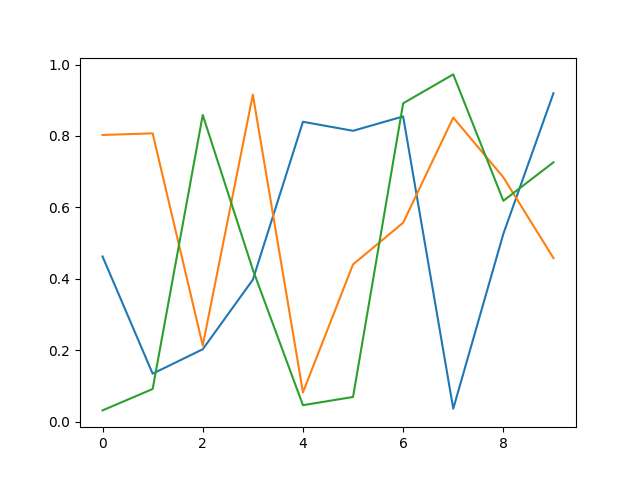which makes three plots, one at a time.

### Summary¶

In interactive mode, pyplot functions automatically draw to the screen.

When plotting interactively, if using object method calls in addition to pyplot functions, then call `draw()` whenever you want to refresh the plot.

Use non-interactive mode in scripts in which you want to generate one or more figures and display them before ending or generating a new set of figures. In that case, use `show()` to display the figure(s) and to block execution until you have manually destroyed them.

## Performance¶

Whether exploring data in interactive mode or programatically saving lots of plots, rendering performance can be a painful bottleneck in your pipeline. Matplotlib provides a couple ways to greatly reduce rendering time at the cost of a slight change (to a settable tolerance) in your plot’s appearance. The methods available to reduce rendering time depend on the type of plot that is being created.

### Line segment simplification¶

For plots that have line segments (e.g. typical line plots, outlines of polygons, etc.), rendering performance can be controlled by the `path.simplify` and `path.simplify_threshold` parameters in your `matplotlibrc` file (see Customizing matplotlib for more information about the `matplotlibrc` file). The `path.simplify` parameter is a boolean indicating whether or not line segments are simplified at all. The `path.simplify_threshold` parameter controls how much line segments are simplified; higher thresholds result in quicker rendering.

The following script will first display the data without any simplification, and then display the same data with simplification. Try interacting with both of them:

```import numpy as np
import matplotlib.pyplot as plt
import matplotlib as mpl

# Setup, and create the data to plot
y = np.random.rand(100000)
y[50000:] *= 2
y[np.logspace(1, np.log10(50000), 400).astype(int)] = -1
mpl.rcParams['path.simplify'] = True

mpl.rcParams['path.simplify_threshold'] = 0.0
plt.plot(y)
plt.show()

mpl.rcParams['path.simplify_threshold'] = 1.0
plt.plot(y)
plt.show()
```

Matplotlib currently defaults to a conservative simplification threshold of `1/9`. If you want to change your default settings to use a different value, you can change your `matplotlibrc` file. Alternatively, you could create a new style for interactive plotting (with maximal simplification) and another style for publication quality plotting (with minimal simplification) and activate them as necessary. See Customizing matplotlib for instructions on how to perform these actions.

The simplification works by iteratively merging line segments into a single vector until the next line segment’s perpendicular distance to the vector (measured in display-coordinate space) is greater than the `path.simplify_threshold` parameter.

Note

Changes related to how line segments are simplified were made in version 2.1. Rendering time will still be improved by these parameters prior to 2.1, but rendering time for some kinds of data will be vastly improved in versions 2.1 and greater.

### Marker simplification¶

Markers can also be simplified, albeit less robustly than line segments. Marker simplification is only available to `Line2D` objects (through the `markevery` property). Wherever `Line2D` construction parameter are passed through, such as `matplotlib.pyplot.plot()` and `matplotlib.axes.Axes.plot()`, the `markevery` parameter can be used:

```plt.plot(x, y, markevery=10)
```

The markevery argument allows for naive subsampling, or an attempt at evenly spaced (along the x axis) sampling. See the Markevery Demo for more information.

### Splitting lines into smaller chunks¶

If you are using the Agg backend (see What is a backend?), then you can make use of the `agg.path.chunksize` rc parameter. This allows you to specify a chunk size, and any lines with greater than that many vertices will be split into multiple lines, each of which have no more than `agg.path.chunksize` many vertices. (Unless `agg.path.chunksize` is zero, in which case there is no chunking.) For some kind of data, chunking the line up into reasonable sizes can greatly decrease rendering time.

The following script will first display the data without any chunk size restriction, and then display the same data with a chunk size of 10,000. The difference can best be seen when the figures are large, try maximizing the GUI and then interacting with them:

```import numpy as np
import matplotlib.pyplot as plt
import matplotlib as mpl
mpl.rcParams['path.simplify_threshold'] = 1.0

# Setup, and create the data to plot
y = np.random.rand(100000)
y[50000:] *= 2
y[np.logspace(1,np.log10(50000), 400).astype(int)] = -1
mpl.rcParams['path.simplify'] = True

mpl.rcParams['agg.path.chunksize'] = 0
plt.plot(y)
plt.show()

mpl.rcParams['agg.path.chunksize'] = 10000
plt.plot(y)
plt.show()
```

### Using the fast style¶

The fast style can be used to automatically set simplification and chunking parameters to reasonable settings to speed up plotting large amounts of data. It can be used simply by running:

```import matplotlib.style as mplstyle
mplstyle.use('fast')
```

It is very light weight, so it plays nicely with other styles, just make sure the fast style is applied last so that other styles do not overwrite the settings:

```mplstyle.use(['dark_background', 'ggplot', 'fast'])
```

Total running time of the script: ( 0 minutes 0.192 seconds)

Gallery generated by Sphinx-Gallery